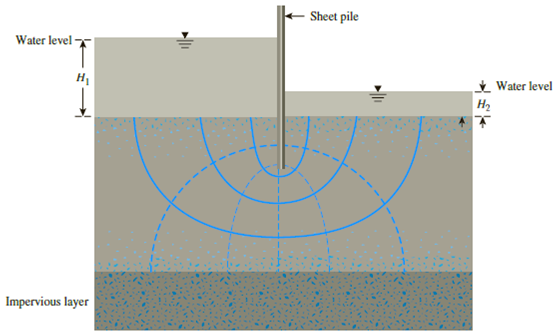Chapter 9, Problem 9.15PPrinciples of Geotechnical Enginee...

9th Edition
Braja M. Das + 1 other
ISBN: 9781305970939

Solutions

Chapter
SectionPrinciples of Geotechnical Enginee...

9th Edition
Braja M. Das + 1 other
ISBN: 9781305970939
Textbook Problem

Determine the factor of safety against heave on the downstream side of the single-row sheet pile structure shown in Figure 9.30. Use the following soil and design parameters: H1 = 7 m, H2 = 3 m, thickness of permeable layer (T) = 12 m, design depth of penetration of sheet pile (D) = 4.5 m, and γsat = 17 kN/m3.Figure 9.30

To determine

Find the factor of safety against heave on the downstream side of the single-row pile sheet structure.

Explanation

Given information:

The water depth in the left side H1 is 7.0 m.

The water depth in the right side H2 is 3.0 m.

The thickness (T) of the permeable layer is 12 m.

The design depth (D) of penetration of sheet pile is 4.5 m.

The saturated unit weight γsat of the soil is 17kN/m3.

Calculation:

Calculate the factor of against heave on the downstream side of the single-row pile sheet structure using the relation.

Factorofsafety=DγCoγw(H1H2) (1)

Here, γ is the submerged unit weight of the soil and Co is the ratio of design depth of penetration of sheet pile to the thickness of the permeable layer.

Determine the submerged unit weight of the soil using the relation.

γ=γsatγwater

Here, γwater is the specific weight of the water.

Take the specific weight of the water as 9.81kN/m3.

Substitute 17kN/m3 for γsat and 9.81kN/m3 for γwater.

γ=179.81=7.19kN/m3

Find the ratio of design depth of penetration of sheet pile to the thickness of the permeable layer.

DT=4.512=0.375

Refer Table (9.1) “Variation of Co with D/T” in the text book.

Take the value of x1 as 0.3.

Take the value of x3 as 0

Still sussing out bartleby?

Check out a sample textbook solution.

See a sample solution

The Solution to Your Study Problems

Bartleby provides explanations to thousands of textbook problems written by our experts, many with advanced degrees!

Get Started

What are the major outdoor air pollutants?

Engineering Fundamentals: An Introduction to Engineering (MindTap Course List)

What do you think are the hallmarks of a good strategic plan?

Principles of Information Systems (MindTap Course List)

What s the Hawthorne Effect? Have you ever experienced it? When and where?

Systems Analysis and Design (Shelly Cashman Series) (MindTap Course List)

Identify risks when using payment apps.

Enhanced Discovering Computers 2017 (Shelly Cashman Series) (MindTap Course List)

What is the purpose of postheating the metal after welding?

Welding: Principles and Applications (MindTap Course List)